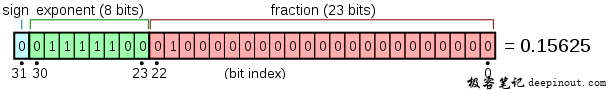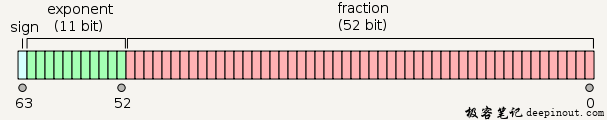# C语言 变量

char 通常是一个字节（八位）。这是一个整数类型。
int 对机器而言，整数的最自然的大小。
float 单精度浮点值。单精度是这样的格式，1位符号，8位指数，23位小数。double 双精度浮点值。双精度是1位符号，11位指数，52位小数。void 表示类型的缺失。

C 语言也允许定义各种其他类型的变量，比如枚举、指针、数组、结构、共用体等等，这将会在后续的章节中进行讲解，本章节我们先讲解基本变量类型。

## C 语言变量定义

``````type variable_list;
``````

``````int    i, j, k;
char   c, ch;
float  f, salary;
double d;
``````

int i, j, k; 声明并定义了变量 i、j 和 k，这指示编译器创建类型为 int 的名为 i、j、k 的变量。

``````type variable_name = value;
``````

``````extern int d = 3, f = 5;    // d 和 f 的声明与初始化
int d = 3, f = 5;           // 定义并初始化 d 和 f
byte z = 22;                // 定义并初始化 z
char x = 'x';               // 变量 x 的值为 'x'
``````

## C 语言变量声明

• 1、一种是需要建立存储空间的。例如：int a 在声明的时候就已经建立了存储空间。
• 2、另一种是不需要建立存储空间的，通过使用extern关键字声明变量名而不定义它。 例如：extern int a 其中变量 a 可以在别的文件中定义的。
• 除非有extern关键字，否则都是变量的定义。
``````extern int i; //声明，不是定义
int i; //声明，也是定义
``````

## C 语言变量实例

``````#include <stdio.h>

// 函数外定义变量 x 和 y
int x;
int y;
{
// 函数内声明变量 x 和 y 为外部变量
extern int x;
extern int y;
// 给外部变量（全局变量）x 和 y 赋值
x = 1;
y = 2;
return x+y;
}

int main()
{
int result;

printf("result 为: %d",result);
return 0;
}
``````

``````result 为: 3
``````

``````#include <stdio.h>
/*外部变量声明*/
extern int x ;
extern int y ;
{
return x+y;
}
``````

test.c 文件代码：

``````/*定义两个全局变量*/
int x=1;
int y=2;
int main(void)
{
int result;
printf("result 为: %d\n",result);
return 0;
}
``````

``````\$ gcc addtwonum.c test.c -o main
\$ ./main
result 为: 3
``````

## C 语言的左值（Lvalues）和右值（Rvalues）

C 中有两种类型的表达式：

1. 左值（lvalue）：指向内存位置的表达式被称为左值（lvalue）表达式。左值可以出现在赋值号的左边或右边。
2. 右值（rvalue）：术语右值（rvalue）指的是存储在内存中某些地址的数值。右值是不能对其进行赋值的表达式，也就是说，右值可以出现在赋值号的右边，但不能出现在赋值号的左边。

``````int g = 20;
``````

``````10 = 20;
``````

• 回顶